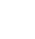# Project: Split Countries into new file

The project brief was to create a macro on a button that would follow this process:

Open dialog box so user can choose file to work with
Identify which column is headed ‘Country’
Take each ‘Country’ by its value (UK, ES, USA etc) in the column and split them into their own file saved in the same location as the users file, copying the entire row over with the header too.

This code uses several features and a function that can be used in part or in full for a similar project.

``` Function SheetExists(SheetName As String, Optional wb As Excel.Workbook) Dim s As Excel.Worksheet If wb Is Nothing Then Set wb = ThisWorkbook On Error Resume Next Set s = wb.Sheets(SheetName) On Error GoTo 0 SheetExists = Not s Is Nothing End Function ```

``` Sub Split_to_new_files_by_value_in_col_Headed_Country() Application.ScreenUpdating = False Application.DisplayAlerts = False```

``` Dim xWs As Worksheet Application.ScreenUpdating = False Application.DisplayAlerts = False For Each xWs In Application.ActiveWorkbook.Worksheets If xWs.Name <> "Main" Then xWs.Delete End If Next Application.DisplayAlerts = True Application.ScreenUpdating = True 'Get name of current file Thswkb = ActiveWorkbook.Name 'Open the dialog box to open a file Application.DisplayAlerts = True NewFN = Application.GetOpenFilename(FileFilter:="Excel Files (*.xls*), *.xls*", Title:="Please select a file") If NewFN = False Then ' They pressed Cancel MsgBox "Choose file cancelled" Exit Sub Else Workbooks.Open Filename:=NewFN End If 'Store the name of the input file opened input_fname = ActiveWorkbook.Name With ActiveSheet Worksheets(1).Copy After:=Workbooks(Thswkb).Sheets(1) End With 'rename the new sheet as Data_IN ActiveSheet.Name = "Data_IN" 'Close the file we took the data from Application.DisplayAlerts = False Workbooks(input_fname).Activate ActiveWorkbook.Close 'Find out the column that has the country code down it cntrycol = WorksheetFunction.Match("Country", Worksheets("Data_IN").Range("1:1"), 0) With Worksheets("Data_IN") lastrow = .Cells(.Rows.Count, "A").End(xlUp).Row End With For n = 2 To lastrow x = Worksheets("Data_IN").Cells(n, cntrycol) If SheetExists("" & x) = True Then GoTo NJB End If Application.DisplayAlerts = False ActiveWorkbook.Sheets.Add Before:=Worksheets(Worksheets.Count) ActiveSheet.Name = x Application.DisplayAlerts = True NJB: Next n 'Loop through the data again and move the row to the correct tab 'But first we put a header in by copying row 1 of Data_IN ShCount = ThisWorkbook.Sheets.Count - 1 'Loop through them from 2 to ShCount-1 For shts = 2 To ShCount Worksheets("Data_IN").Range("A1").EntireRow.Copy Destination:=Worksheets(shts).Range("A1") Next shts 'Go down the data again, grabbing country code With Worksheets("Data_IN") lastrow = .Cells(.Rows.Count, "A").End(xlUp).Row End With For p = 2 To lastrow x = Worksheets("Data_IN").Cells(p, cntrycol) With Worksheets(x) lastrowX = .Cells(.Rows.Count, "A").End(xlUp).Row End With Worksheets("Data_IN").Range("A" & p).EntireRow.Copy Destination:=Worksheets(x).Range("A" & lastrowX + 1) Next p 'Now save each sheet except first and last as the inout name then the sheet name real_name_extns = InStr(1, input_fname, ".") - 1 real_name = Left(input_fname, real_name_extns) & "_" Application.DisplayAlerts = False For wshts = 2 To ShCount Sheets(wshts).Activate i = ActiveSheet.Name ActiveSheet.Copy With ActiveWorkbook .SaveAs ThisWorkbook.Path & "\" & real_name & i & ".xlsx" .Close End With Next wshts Worksheets("Main").Select MsgBox "Completed Country Split" ```

```End Sub ```